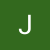# The Blind 75 Leetcode Series: Maximum Depth of Binary TreePhoto by Chris Ried on Unsplash

Today, we are working on 104. Maximum Depth of Binary Tree

Given the `root` of a binary tree, return its maximum depth.

A binary tree’s maximum depth is the number of nodes along the longest path from the root node down to the farthest leaf node.

We have a tree, and we want to find how many levels down is the tree. We want to return the max depth of this tree.

We can actually use something similar to the Binary Level Tree Traversal problem. We traverse the tree and record the level. For that problem, we need to keep tracking the level and the node belonging to the level. For this problem, we only care about the level. At each node, we compare the current level and the max level we record, and choose the larger one. When we are done traversing the tree, we simply return the max depth.

Following the concept in the Binary tree traversal problem, we create a recursive function taking the node, the current depth, and, instead of the tracker we used to track each level’s nodes, a simple integer tracking the max depth.

We first compare the current depth and the max depth. Store the larger one. Then we check if this node has any child, if not, return the max_depth. If yes, recursively call the same function with level = level +1.

The recursive function would look like this:

`def checkDepth(root, depth, max_depth):    max_depth = max(max_depth, depth)    if not root:        return max_depth    if root.left:        max_depth = checkDepth(root.left, depth + 1, max_depth)    if root.right:        max_depth = checkDepth(root.right, depth + 1, max_depth)    return max_depth`

And then for the top level, we simply pass in `1` for current_level and `1` for max_level. We can also check if the tree is empty. If so, return `0`.

The overall script looks like this:

`def checkDepth(root, depth, max_depth):    max_depth = max(max_depth, depth)    if not root:        return max_depth    if root.left:        max_depth = checkDepth(root.left, depth + 1, max_depth)    if root.right:        max_depth = checkDepth(root.right, depth + 1, max_depth)    return max_depthdef maxDepth(root):    if not root:        return 0    max_depth = checkDepth(root, 1, 1)    return max_depth`

What’s the time complexity? We are traversing through the entire tree, so if the tree has N nodes, we are looking at O(N) for overall time complexity.

This is once again a DFS approach. Can we achieve the same result with a BFS?

For BFS, we need a queue to store the nodes. Let’s use `deque` so we can easily pop from the beginning of the queue.

We then need to store the node and its level as a tuple into the queue. Every time we get a node from the queue, we compare the level with max_depth, then we check for left and right children and store the children in the queue.

We continue until we run out of node to check in the queue, then we just return the max_depth

The code will look like this:

`def maxDepth(root):    if not root:        return 0    queue = deque()    queue.append((root, 1))    max_depth = 1    while queue:        node, current_level = queue.popleft()        max_depth = max(max_depth, current_level)        if node.left:            queue.append((node.left, current_level + 1))        if node.right:            queue.append((node.right, current_level + 1))    return max_depth`

The time complexity is once again O(N) as we traverse through the entire tree.

Once again, be sure to know when and how to use DFS and BFS and what’s required.

That’s it! Another problem down.